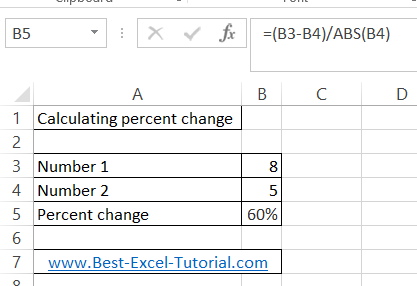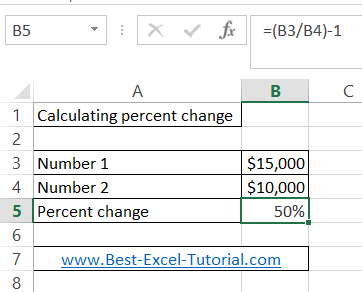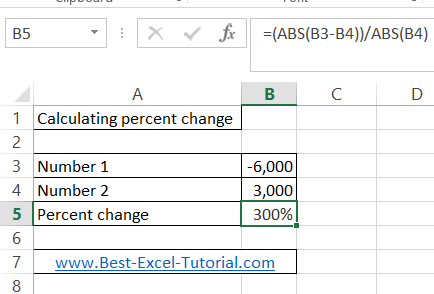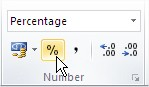#### How to Calculate Percentage Change

Percentage change in Excel is used to determine the difference between two values as a percentage. This can be useful for comparing changes in financial data, such as sales or profits, over time. In this lesson you will learn, how to calculate the change between two numbers.

## Calculating Percentage Change in Excel

Calculating percent change with these 2 formulas is really simple in Excel:

• =(new_value/original_value)-1
• =ABS(new_value-original_value)/ABS(original_value)

= (new_value/original_value) – 1 is for beginners. This is a really simple formula with which you can calculate the percent change of two numbers. Remember that this syntax will give you a wrong result when one of the numbers is less than zero.

=ABS(new_value-original_value)/ABS(original_value) is more difficult, but it gives always correct results. I suggest you only use the second formula.

The ABS function returns the absolute value of a number. The absolute value of a number is the number without its sign.

Syntax: =ABS(number)

Number is the real number of which you want the absolute value.

## Examples

### Example 1 –  using ABS function to calculate percent change

Number1: 8

Number2: 5

Formula is: =(8/5)-1

or

Formula is: =(8-5)/ABS(5)Result is: 60%

Full answer: 8 is 60% greater than 5.

### Example 2 – simple way to calculate percent change

Number1: \$15 000

Number2: \$10 000

Formula is: =(15000/1000)-1

or

Formula is: =(15000-10000)/ABS(10000)Result is: 50%

Full answer: \$15 000 is 50% greater than 10 000.

### Example 3 – more difficult way of calculation percent change

This example is more difficult because one of the numbers is below zero. Thus you can use only the second formula with ABS function.

Number1: -6 000

Number2: 3 000

Formula is: =(-6000-3000)/ABS(3000)Result is: 300%

Full answer: -6 000 is 300% less than 3 000.

Using such a kind of formula will always give you the proper result. No matter if any of the numbers are below zero. To calculate percent change in Excel, I recommend only using this formula.

You can also use the Excel percentage formula, which is =CHANGE(A1, B1). This formula calculates the percentage change between two values. For example, if the original value is 100 and the new value is 120, the result of the formula would be 20%.

## Tip – percent style formatting

To format Percent Style cell just go to Ribbon > Home > Number and click Percent Style button.